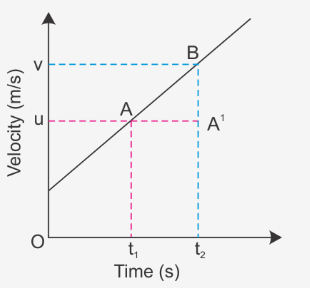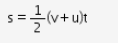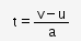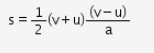# Derive the third equation of motion v2 - u2 = 2as using velocity-time graph

Derive the third equation of motion v2 - u2 = 2as using velocity-time graph.

Consider the velocity-time graph for a body having some non zero initial velocity at time t = 0.u: velocity at time t1
v: velocity at time t2
a: uniform acceleration of the body along the straight line

Displacement covered during the time interval t2 - t1 = Area ABt1 t2
S = Area of triangle ABA’ + Area of rectangle AA’t2 t1 = Area of trapezium
S = ½ x Sum of parallel sides x Perpendicular distance… (Equation 1)
From the first equation of motion, v = u + at;Substituting in equation 1, we getor, v2 – u2 = 2as
which is the required equation of motion.